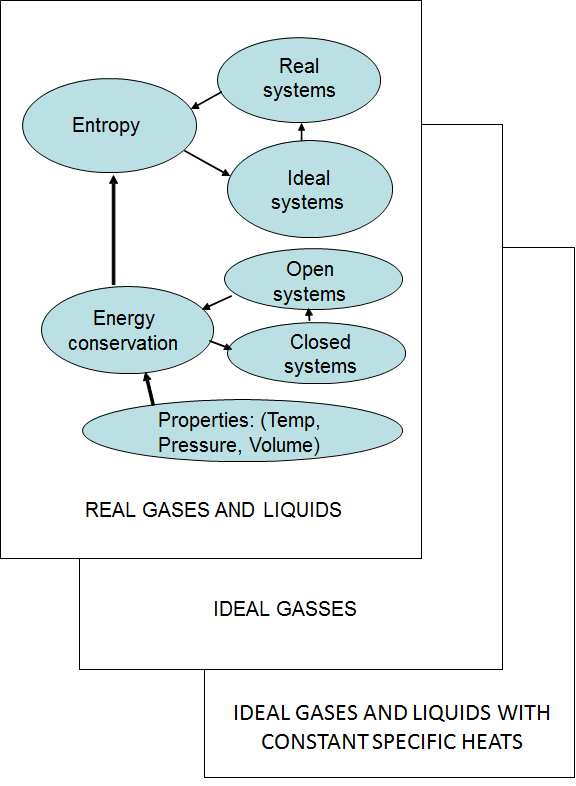# Chapter 1 Preface

Thermodynamics is the study of energy and energy conversion. For example, in a coal fired power station, during the combustion of coal, the energy in the coal is released as heat that is used to convert high pressure liquid water into high pressure steam. In a turbine the energy in the steam is converted into mechanical energy which turns the shaft of the turbine in order to generate electric energy in the generator. Often this electric energy is again converted into heat in the stove plate of an electric stove or the heating element of a geyser. In a motorcar the energy in the fuel is converted into mechanical energy to turn the wheels of the car.

With the invention of the steam engine, an interest arose in understanding the scientific principles which lay behind these enginese in order to improve their efficiency. Heat was seen as a fluid (caloric) that flows from a high temperature to a low temperature and that heat and work were unrelated. It was discovered that heat and work are both forms of energy and that energy cannot be created or destroyed. This led to the formulation of the First Law of Thermodynamics which states that energy is not created or destroyed but is merely converted from one form to another. The Second Law of Thermodynamics was also formulated. One formulation states that on a molecular level, order in the universe keeps on decreasing. This decrease is associated with a decrease in the ability to do work. This means that while the amount of energy before and after a conversion process is the same, the ability of the energies to do work, have decreased during the conversion. This correlates with our everyday experience. Driving in a circle with a car, the fuel powers the wheels of the car but eventually all the energy in the fuel is converted to low temperature heat due to friction and turbulence.

Thermodynamics is good example of an engineering science. Engineering sciences differ from the natural sciences such as physics and chemistry. Hansson (2007) identified a few characteristics of engineering sciences:

• A focus on the human-made objects rather than natural objects. Turbines rather than tornadoes;

• Do not require exact (often analytical) solutions but are satisfied with solutions that are sufficiently accurate (often numerical);

• Utilization of restricted idealizations. Because technological objects need to function in the real world, idealizations that are useful in the natural sciences can often not be made in engineering sciences.

Students can find textbooks in Thermodynamics quite intimidating. The rigorous derivation of the laws and equations can be quite complex with many abstract concepts introduced simultaneously. No wonder Thermodynamics is perceived as difficult. In this document an effort is made to introduce concepts sequentially and develop understanding gradually in an logical and concrete manner. It is a first, introductory text.

The logical way to explain the conservation of energy is to first cover closed systems (the control mass) combining heat, work and internal energy in the First Law. Using that as foundation, the concept of enthalpy is developed and the conservation of energy in open systems (control volumes) explained. Once the student understands the concept of energy conservation, a solid foundation for understanding reversibility and entropy has been laid - be it in open or closed systems. Once students are comfortable with reversibility and entropy they should find it easier to understand real systems and the generation of entropy. Therefore, the Second Law is covered last. The approach followed in this text is shown schematically in Figure 1.1 below.Figure 1.1: Overview

In the rest of the text, an effort is again made to introduce concepts one at a time. For instance, instead of including potential and kinetic energy during the introduction of the First Law for closed systems, and then ignoring them again during most (if not all) subsequent implementations, initially only heat, work and internal energy are considered. Once the student has had ample opportunity to master the concept of energy conservation, systems where kinetic and potential energy are relevant, are discussed.